## 直接複寫預設顯示格式

1. 在 Views –> Shared 資料夾中新增 `DisplayTemplates` 資料夾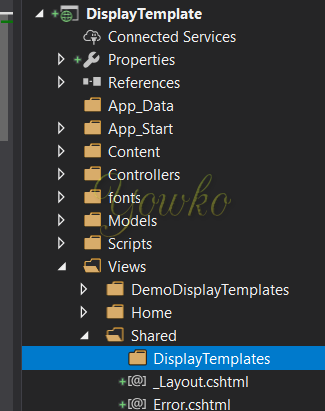2. `DisplayTemplates` 資料夾建立以 `目標資料型態` (e.g. `DateTime``Decimal`) 為名稱的 View

使用時不需另外編譯

• DateTime

• 加入 `DateTime.cshtml`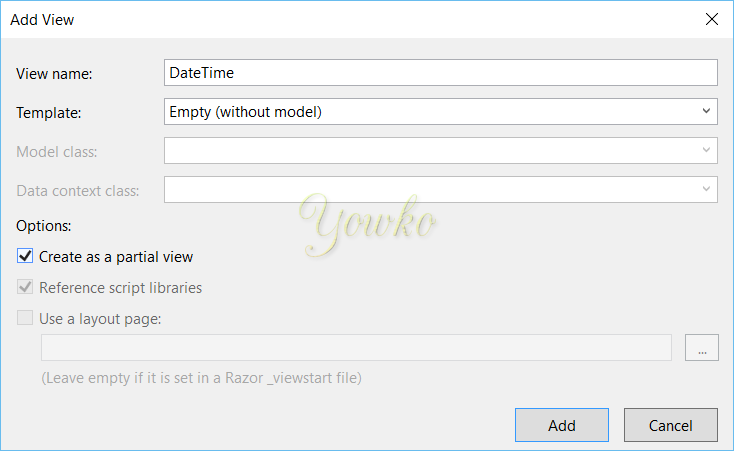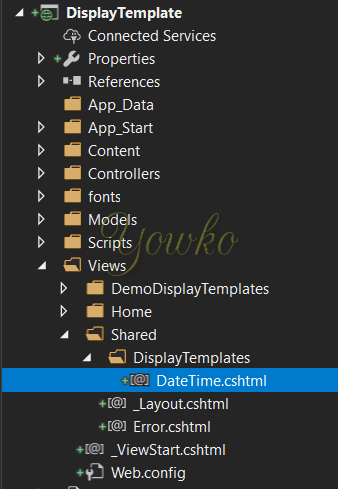• 指定 model 型態(記得處理 null)

``````@model DateTime?
``````
• 未處理 null 錯誤

``````The model item passed into the dictionary is null, but this dictionary requires a non-null model item of type 'System.DateTime'.
``````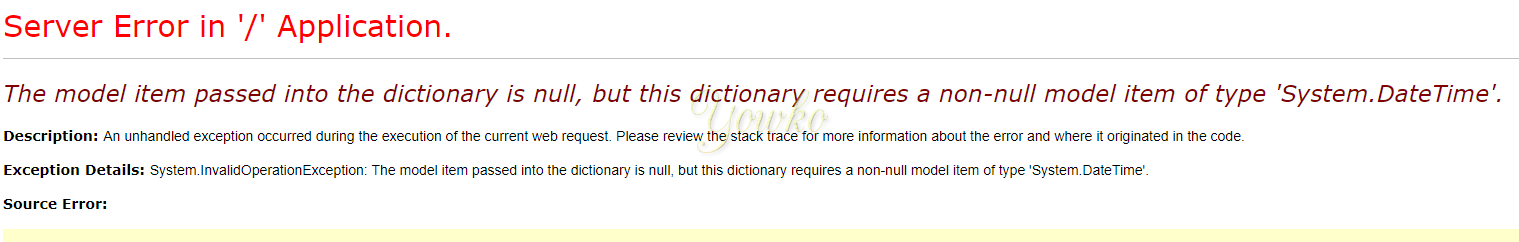• 依實際需求客製輸出格式

``````@if (Model == null)
{
<span class="text-danger">No DateTime Data!!</span>
}
else
{
<span>@(((DateTime)Model).ToString("MM/dd/yyyy HH:mm:ss"))</span>
}
``````
• 完整程式碼

``````@model DateTime?
@if (Model == null)
{
<span class="text-danger">No DateTime Data!!</span>
}
else
{
<span>@(((DateTime)Model).ToString("MM/dd/yyyy HH:mm:ss"))</span>
}
``````
• 前後差異

• 修改前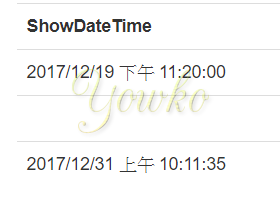• 修改後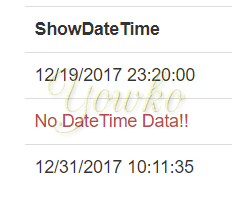• Decimal

• 加入 `Decimal.cshtml`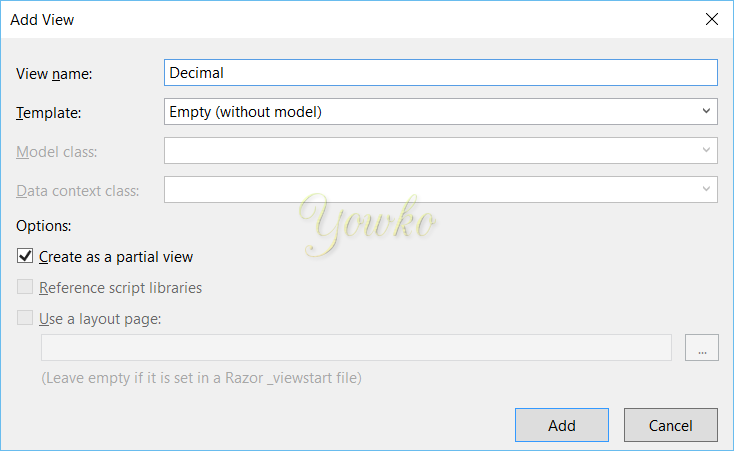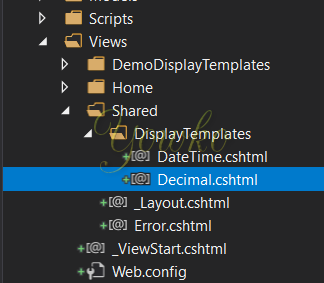• 無法加入 `decimal.cshtml`(可以加入 `Decimal.cshtml` 後再 rename)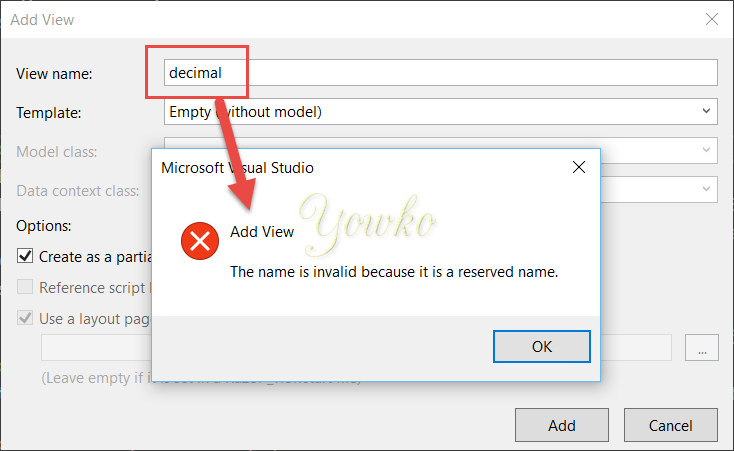• 指定 model 型態(記得處理 null)

``````@model decimal?
``````
• 未處理 null 錯誤

``````The model item passed into the dictionary is null, but this dictionary requires a non-null model item of type 'System.Decimal'.
``````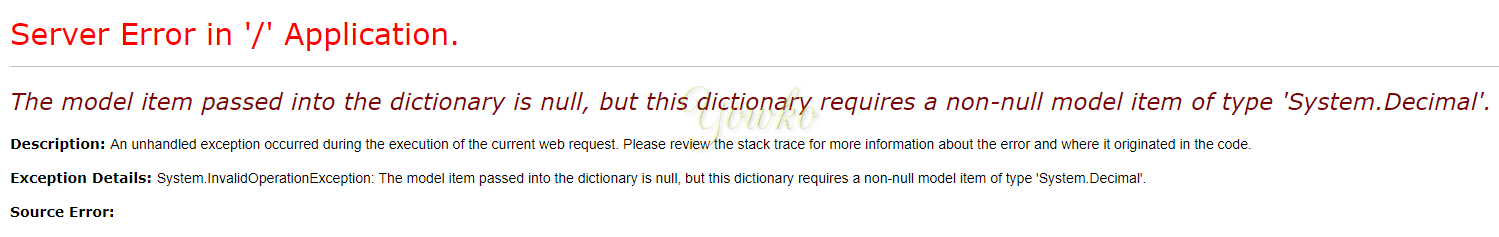• 依實際需求客製輸出格式

``````@if (Model == null)
{
<span class="text-danger">No Decimal Data!!</span>
}
else
{
<span>@(((decimal)Model).ToString("N"))</span>
}
``````
• 完整程式碼

``````@model decimal?

@if (Model == null)
{
<span class="text-danger">No Decimal Data!!</span>
}
else
{
<span>@(((decimal)Model).ToString("N"))</span>
}
``````
• 前後差異

• 修改前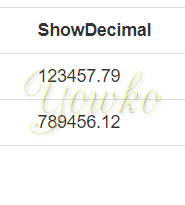• 修改後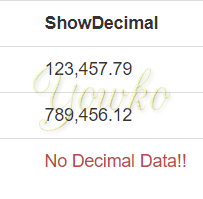3. 使用上檔案名稱不分大小寫

• `DateTime.cshtml` 等於 `datetime.cshtml`
• `Decimal.cshtml` 等於 `decimal.cshtml`

## 額外新增顯示格式

• 新增方式與上述做法相同

• 在 Views –> Shared 新增 `DisplayTemplates` 資料夾• `DisplayTemplates` 資料夾建立 View (View 名稱不能與資料型態相同 - 會直接複寫預設格式)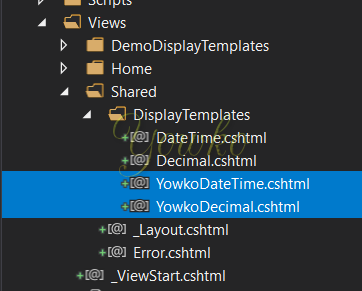1. 手動指定

• 格式

``````@Html.DisplayFor(modelItem => modelItem.{欄位},"{自訂 View 名稱}")
``````
• 實例

``````@Html.DisplayFor(modelItem => item.ShowDateTime,"YowkoDateTime")
``````
• 自訂程式碼(`YowkoDateTime.cshtml`)

``````@model DateTime?
@if (Model == null)
{
<span class="text-danger">Yowko:No DateTime Data!!</span>
}
else
{
<span>Yowko:@(((DateTime)Model).ToString("MM/dd/yyyy HH:mm:ss"))</span>
}
``````
• 效果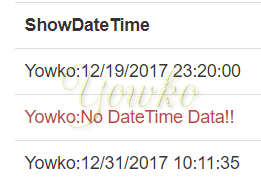2. 使用 UIHint attibute

• 格式

``````[UIHint("{自訂 View 名稱}")]
public type name { get; set; }
``````
• 實例

``````[UIHint("YowkoDecimal")]
public Nullable<decimal> ShowDecimal { get; set; }
``````
• 自訂程式碼(`YowkoDecimal.cshtml`)

``````@model decimal?

@if (Model == null)
{
<span class="text-danger">Yowko:No Decimal Data!!</span>
}
else
{
<span>Yowko:@(((decimal)Model).ToString("N"))</span>
}
``````
• 效果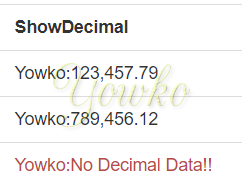• 缺點：因為改到 .cs 需要 compile

## 參考資訊

1. Display templates